# Quantification of resolution

## Mesh resolution

The grid resolution corresponds to uniform $\Delta x^{+}=12.2,\ \Delta z^{+}=6.3$and ranges from $\Delta y^{+}=0.16$near the wall (first solution point) to $\Delta y^{+}=4.6$at the centre of the channel. The near-wall Kolmogorov length scale, $\eta =\left({\bar {\rho }}\nu ^{3}/\epsilon \right)^{1/4}$, in wall units is $\eta ^{+}=1.54$.

## Solution verification

The solution accurately captures the near wall and log-law behaviour of averaged streamwise velocity profile as shown in Fig. 2.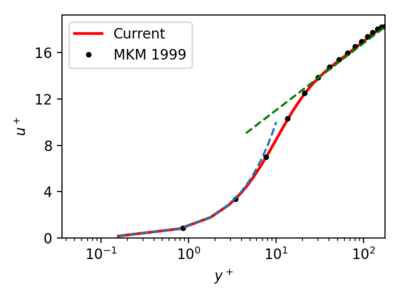Figure 3: Average streamwise velocity, $Re_{\tau }=180$, vs data from Moser et al. (1999)

The turbulent stress profiles, shown by the solid lines, and the turbulent kinetic energy profile, represented by the dashed line, in figure 4 agree well with the data from Moser et al., which is indicated by the markers.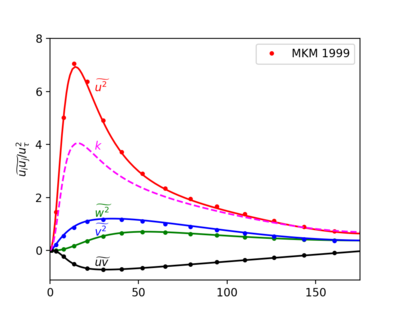Figure 4: Turbulent stress profiles

The expected near wall behaviour of turbulent stresses are ${\widetilde {u^{\prime \prime 2}}},{\widetilde {w^{\prime \prime 2}}}\sim y^{2},\ {\widetilde {v^{\prime \prime 2}}}\sim y^{4}$and ${\widetilde {u^{\prime }\prime v^{\prime }\prime }}\sim y^{3}$. These stresses near the wall from the current set of simulations are shown below.

The turbulent kinetic energy budget (Fig. 6), as well as budgets of the diagonal components of the turbulent stress tensor (fig. 7-9) across the channel, is compared with data from Moser et al. The budget of $R_{uv}$, the shear stress responsible of turbulent energy production is also shown (fig. 10). The budget quantities are non-dimensionalized using $u_{\tau }^{3}/\delta _{\nu }$. The lines represent data from the current set of simulations, compared against Moser's data represented by circles. The dashed black line denotes the residual of the budget. The legend shown in the turbulent kinetic energy budget applies to all the budget plots. The green line in the turbulent kinetic energy budget represents the trace of the pressure-strain tensor, known as pressure-dilatation. In other budget plots, the green line is for corresponding pressure-strain correlation.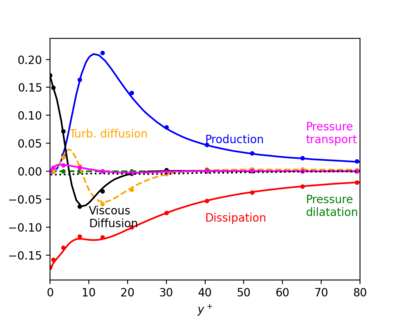Figure 6: Turbulent kinetic energy budget.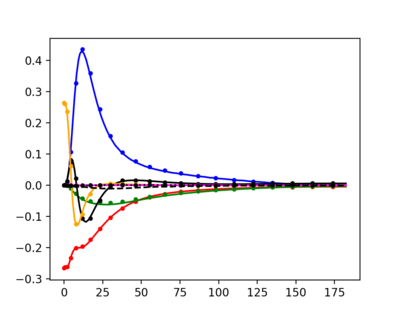Figure 7: Budget of the normal stress component, ${\widetilde {u^{\prime \prime 2}}}$.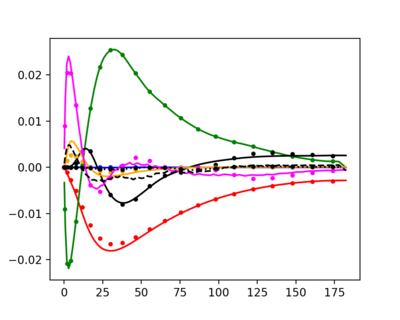Figure 8: Budget of the normal stress component, ${\widetilde {v^{\prime \prime 2}}}$.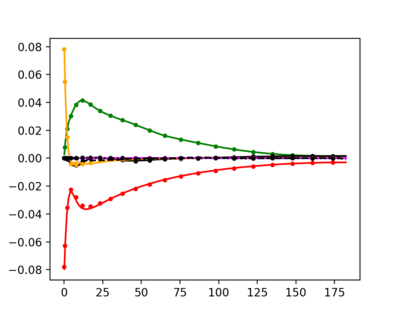Figure 9: Budget of the normal stress component, ${\widetilde {w^{\prime \prime 2}}}$.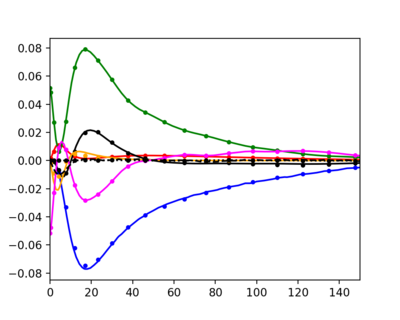Figure 10: Budget of turbulent shear stress, ${\widetilde {u^{\prime \prime }v^{\prime \prime }}}$.

Contributed by: Arun Soman Pillai, Peter Vincent — Imperial College London